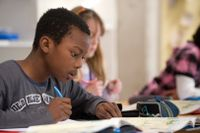George Lucas Educational Foundation
Critical Thinking

Getting to the Heart of What Students Know in Math

Challenging problems with several paths to the solution encourage students to develop their understanding of mathematical concepts.

December 26, 2019Agencja Fotograficzna Caro / Alamy Stock Photo

Imagine a math problem that could appropriately challenge all of your students, give you the ability to clearly distinguish between what they understand and what they’re struggling with, and provide context for powerful student discussions. To explore what that looks like, let’s compare three problems on subtracting three-digit numbers. Take a few minutes to work on these problems and then continue reading.

Problem One: Solve.

812 – 357 =

Problem Two: Using the digits 1 to 9 at most one time each, place a digit on each underscore to make two three-digit numbers that form a true number sentence. Solve the problem twice; you may reuse digits from the first sentence in the second sentence. (I recommend not allowing students to use 0 in these kinds of problems to prevent them from using a leading 0—e.g., 091 = 91.)

_ _ _ – 291 = _ _ _

Problem Three: Using the digits 1 to 9 at most one time each, place a digit on each underscore to make a difference that is as close to 329 as possible.

_ _ _ – _ _ _ = _ _ _

Problem One is a fairly typical problem for subtracting three-digit numbers. If a student gets this problem wrong, we know that’s not good. However, if she gets it right, does it guarantee that she deeply understands the concept? We’ve all had students who appeared to understand a concept, only to find out later that they had hidden misconceptions. So when a student gets this right, it may not guarantee deep understanding.

Problem Two is not easy. I made the problem, and it still took me four attempts just to find one number sentence. Picking digits randomly is an inefficient strategy, so students are forced to use conceptual understanding to think about what digits would be better choices to start with. I bet you can picture students who would have no issues with Problem One but would struggle with Problem Two.

Problem Three is really challenging. Even with a calculator it would likely take multiple attempts to solve the problem. Again, if students are randomly picking digits and subtracting them, it’s going to take a really long time to figure out an answer. Ultimately, this problem forces students to move beyond being a human calculator and build conceptual understanding.

For example, I would hope that students realize that the number on the left has to be greater than 329 or the difference will be closer to 0 than to 329. That’s not enough, though. For example, if a student starts with 987, what comes next? Does she randomly guess digits for the number on the right? Perhaps she starts thinking, “Hmm. I guess the number on the right needs to be about 300 less than the number on the left?” Even using that level of conceptual understanding will really help. Alternatively, students might think about Problem Three as an addition problem and think, “What three-digit number plus 329 equals my other three-digit number?” There are many options for how students might approach it.

What Students Get From This Kind of Problem

You may wonder how students handle these three problems, so let me share data collected from hundreds of third graders who have worked on them. You probably won’t be surprised to learn that students had the most success with Problem One, with 62 percent of students correctly solving the problem, while fewer students solved Problems Two and Three correctly—36 percent and 29 percent, respectively.

This suggests that using only problems like Problem One could give us false positive results, which may incorrectly lead us to think that our students understand what we taught them. I wish I had known this earlier in my career, when I used countless problems like Problem One with my students. I’ve come to realize that when we ask superficial questions, we get superficial information back about what students know.

Problems like Two and Three are called Open Middle problems—a term I learned from math educator Dan Meyer—because there are a variety of strategies students can use to solve them. Most math problems begin with everyone working on the same problem and end with them arriving at the same answer—the beginning and ending are closed. What varies is the middle. Sometimes a problem’s instructions tell students to complete a problem using a specific method (closed middle). Other times, there are many possible ways to solve the problem (open middle). Problems with open middles tend to be much more interesting.

These problems work great anywhere you might give students practice doing the same kinds of problems over and over, such as classwork or homework.  As you might imagine, a single Open Middle problem often necessitates similar amounts of repeated practice as an entire worksheet, but with better conversations and deeper understandings. This kind of problem can also be used as an assessment to help you better understand which students are mastering a concept and which are struggling and need your help.

Open Middle problems can reveal students’ hidden misconceptions, help build their conceptual understanding, and lead to great classroom conversations about how different strategies are connected to one another. And kids love doing them. At Open Middle, you can find hundreds of free problems for all grade levels, including hints you can offer students when they get stuck.